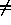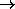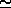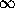# Greek letters and special symbols

A limited number of special characters (such as Greek letters, sub- and superscripts, and a few others) may be indicated in CIF text for typesetting purposes, using the special codes listed below.

## Greek letters

In general, the corresponding letter of the Latin alphabet, prefixed by a backslash character. The complete set is:

 α Α \a \A alpha β Β \b \B beta χ Χ \c \C chi δ Δ \d \D delta ε Ε \e \E epsilon φ Φ \f \F phi γ Γ \g \G gamma η Η \h \H eta ι Ι \i \I iota κ Κ \k \K kappa λ Λ \l \L lambda μ Μ \m \M mu ν Ν \n \N nu ο Ο \o \O omicron π Π \p \P pi θ Θ \q \Q theta ρ Ρ \r \R rho σ Σ \s \S sigma τ Τ \t \T tau υ Υ \u \U upsilon ω Ω \w \W omega ξ Ξ \x \X xi ψ Ψ \y \Y psi ζ Ζ \z \Z zeta

## Accented letters

Accents should be indicated by using the following codes before the letter to be modified (i.e. use \'e for an acute e):

 \' acute (é) \" umlaut (ü) \= overbar \` grave (à) \~ tilde (ñ) \. overdot \^ circumflex (â) \; ogonek \< hacek \, cedilla (ç) \> Hungarian umlaut \( breve

## Other characters

Other special alphabetic characters should be indicated as follows:

 \%a a-ring (å) \?i dotless i \&s German "ss" (ß) \/o o-slash (ø) \/l Polish l () \/d barred d

Capital letters may also be used in these codes, so an ångström symbol (Å) may be given as \%A.

Superscripts and subscripts should be indicated by bracketing relevant characters with circumflex or tilde characters, thus:

 superscripts Csp^3^ for Csp3 subscripts U~eq~ for Ueq

The closing symbol is essential to return to normal text.

Other codes are also recognized by the IUCr software. These are:

 \% degree (°) \\times × -- dash +- ± --- single bond -+\\db double bond \\square square \\tb triple bond \\neq\\ddb delocalized double bond \\rangle\\sim\\langle(N.B. ~  is the code for subscript) \\rightarrow\\simeq\\leftarrow\\inftyNote that \\db, \\tb and \\ddb should always be followed by a space, e.g. C=C is denoted by C\\db C.

Follow Acta Cryst. B